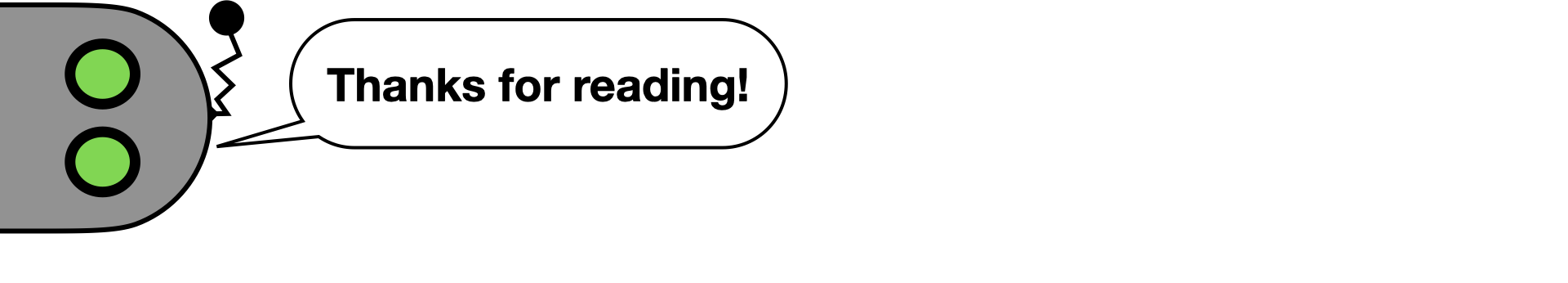# Learning Markov State Abstractions for Deep Reinforcement Learning

Cam Allen – 12 December 2021 – 12 min read

Last week at NeurIPS 2021, I presented a paper with Neev Parikh, Omer Gottesman, and George Konidaris on learning suitable abstract representations for reinforcement learning. We describe novel theoretical conditions for a state abstraction to provably preserve the all-important Markov property, and introduce a practical training objective for approximately learning such an abstraction with deep neural networks—all without requiring reward information, pixel reconstruction, or transition modeling.

[Paper] [Code] [Talk] [Poster] [Bibtex]

## Reinforcement Learning in MDPs

Reinforcement learning (RL) makes the relationship between a decision-making agent and its environment explicit. The decision process is represented as a loop: the agent takes an action, thereby affecting the environment, and subsequently observes the result through its sensors (along with any reward information), at which point the agent selects another action and the process repeats.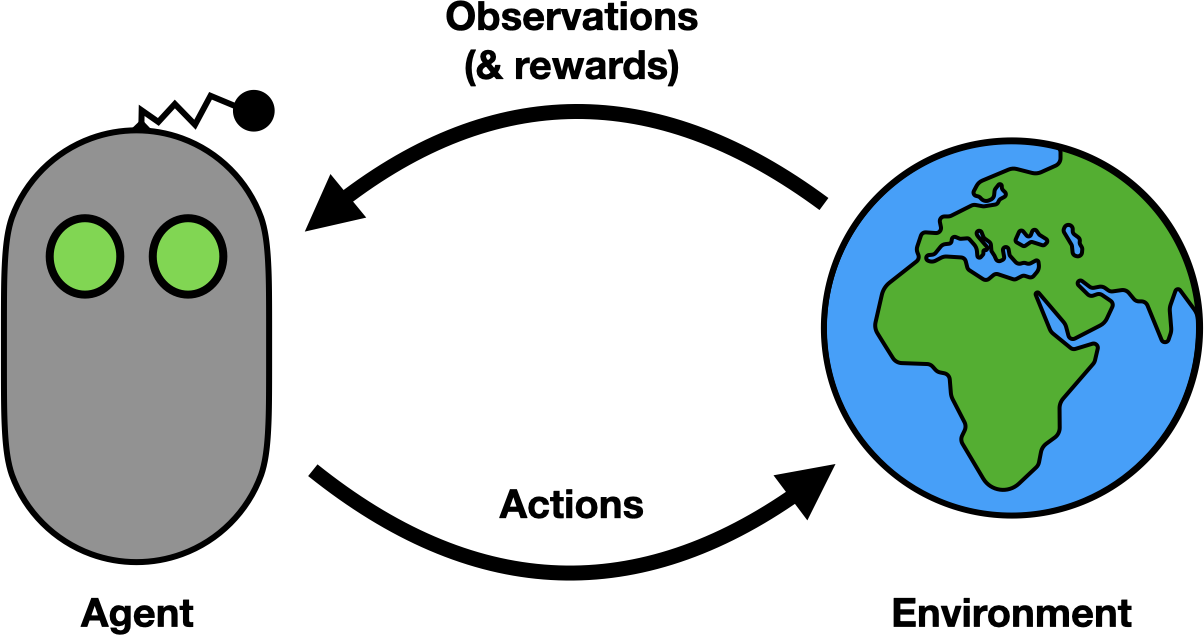The reinforcement learning loop.

RL is an incredibly flexible framework and can easily be customized for specific applications or to encode various types of assumptions or domain knowledge. In this work, we consider the most basic model, the Markov decision process, wherein observations are assumed to contain complete state information about every relevant aspect of the agent-environment system.

Consider an example decision problem involving a robot agent that wants to go to bed.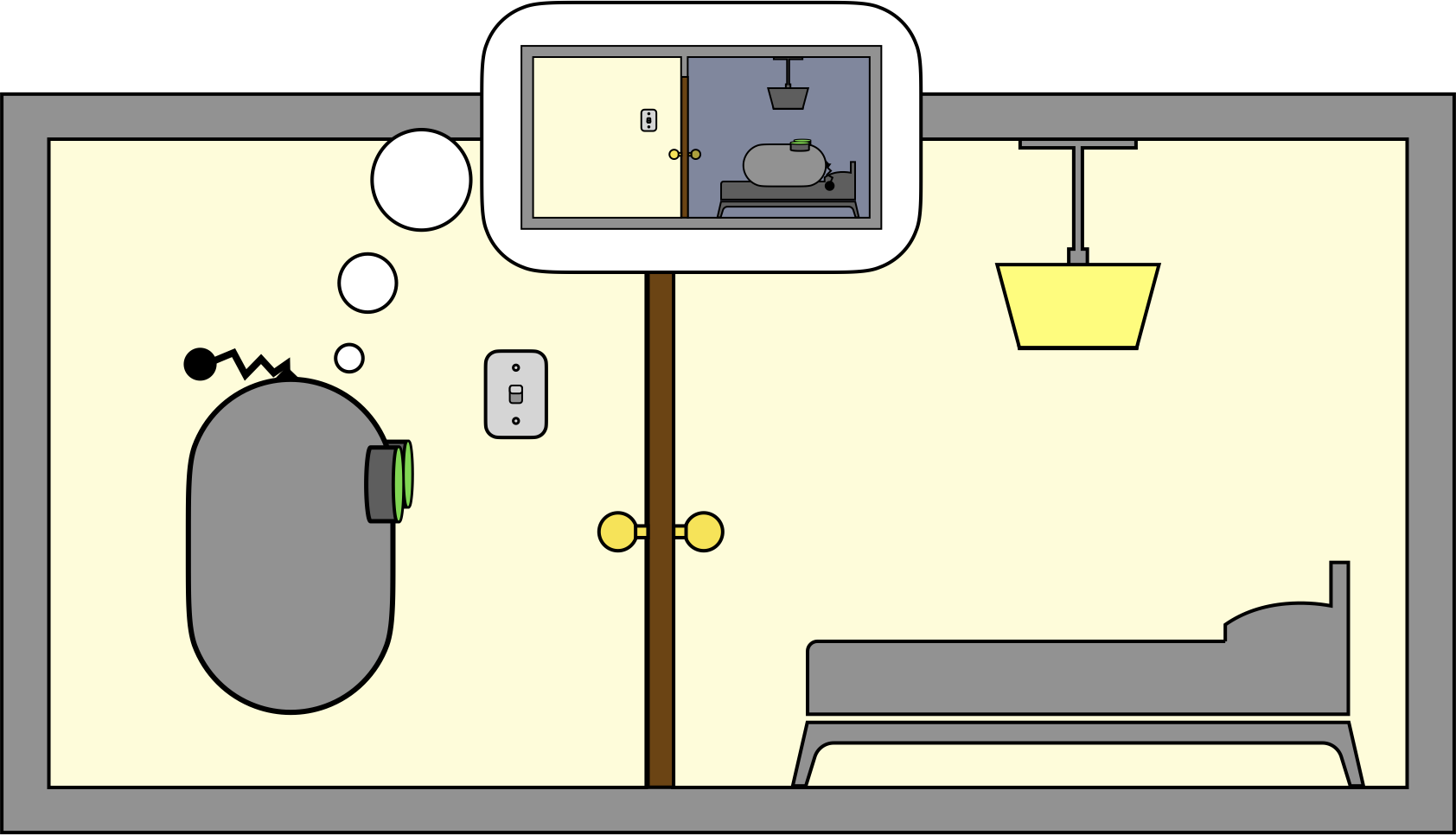The bedtime problem. (Do androids dream of electric sheep?)

Suppose the agent has three actions to choose from: MOVE, TOGGLE_SWITCH, and SLEEP. The MOVE action is applicable anywhere, and it moves the agent from one room to the other. The TOGGLE_SWITCH action changes the state of the bedroom light but is only applicable when the agent is in the hallway (since that is where the lightswitch is). The SLEEP action is only applicable in the bedroom, and the associated reward depends on the state of the bedroom light.

We can visualize the decision problem with the following diagram: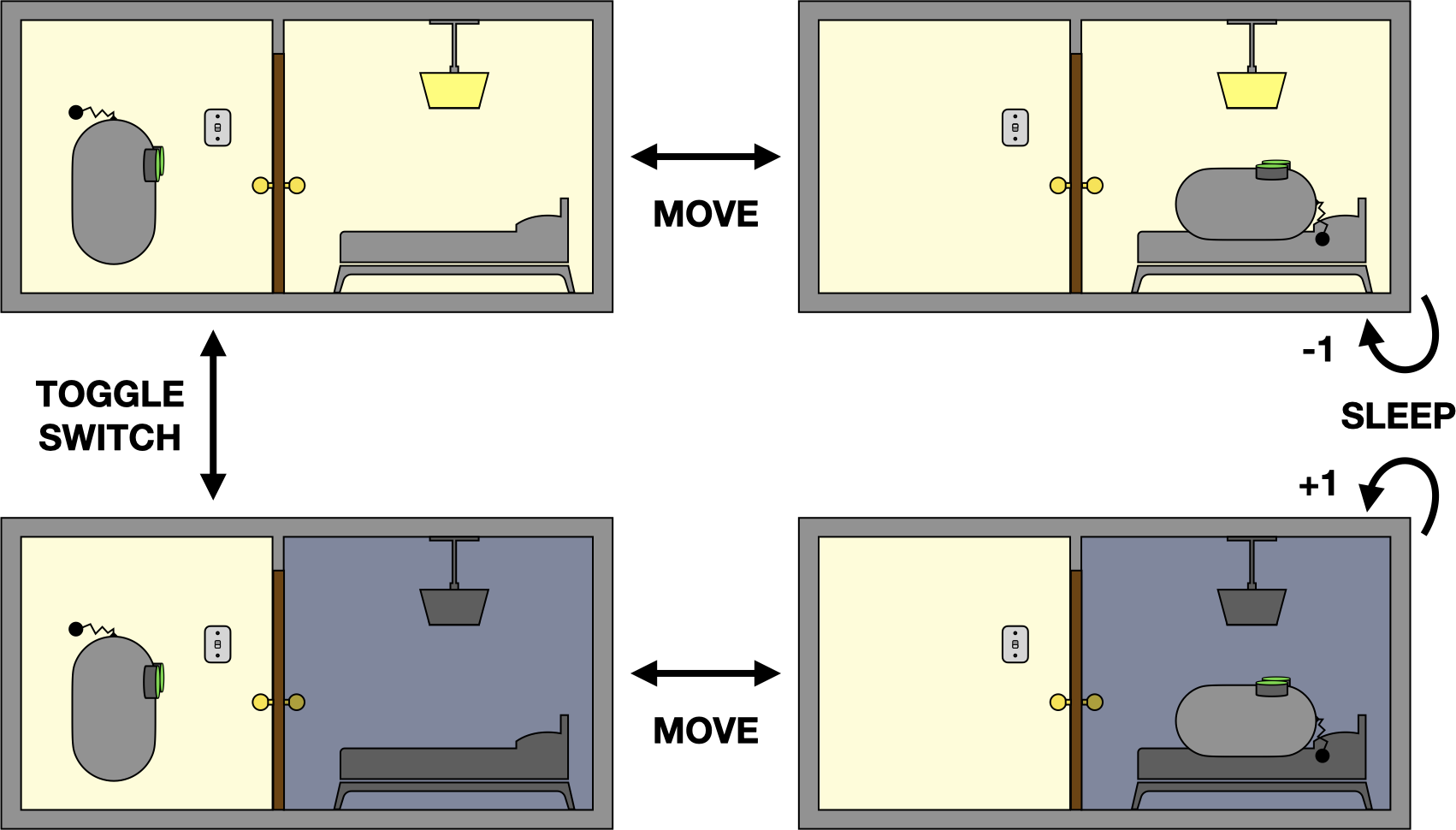An MDP representing the bedtime problem.

Each image in the diagram depicts a possible world state of the agent-environment system, and the arrows depict the applicable actions for each state and their corresponding state transitions. We can imagine that the agent’s preference for going to bed influences the rewards it receives: +1 for trying to sleep with the light off; -1 for trying to sleep with the light on; and zero otherwise.

The optimal agent behavior can be expressed as a policy, a function mapping from states to actions:

• (IN BEDROOM, LIGHT ON): MOVE
• (IN HALLWAY, LIGHT ON): TOGGLE_SWITCH
• (IN HALLWAY, LIGHT OFF): MOVE
• (IN BEDROOM, LIGHT OFF): SLEEP

The key aspect of Markov decision processes, and the one for which they get their name, is that the transition dynamics, rewards, applicable actions, and optimal policy all exhibit the so-called Markov property. The Markov property says that knowing the current world state is enough to fully characterize the system—no additional information is required for making decisions.

To illustrate why the Markov property is desirable, suppose we had instead drawn the following diagram, where each image now depicts the agent’s (partial) observation of the world state: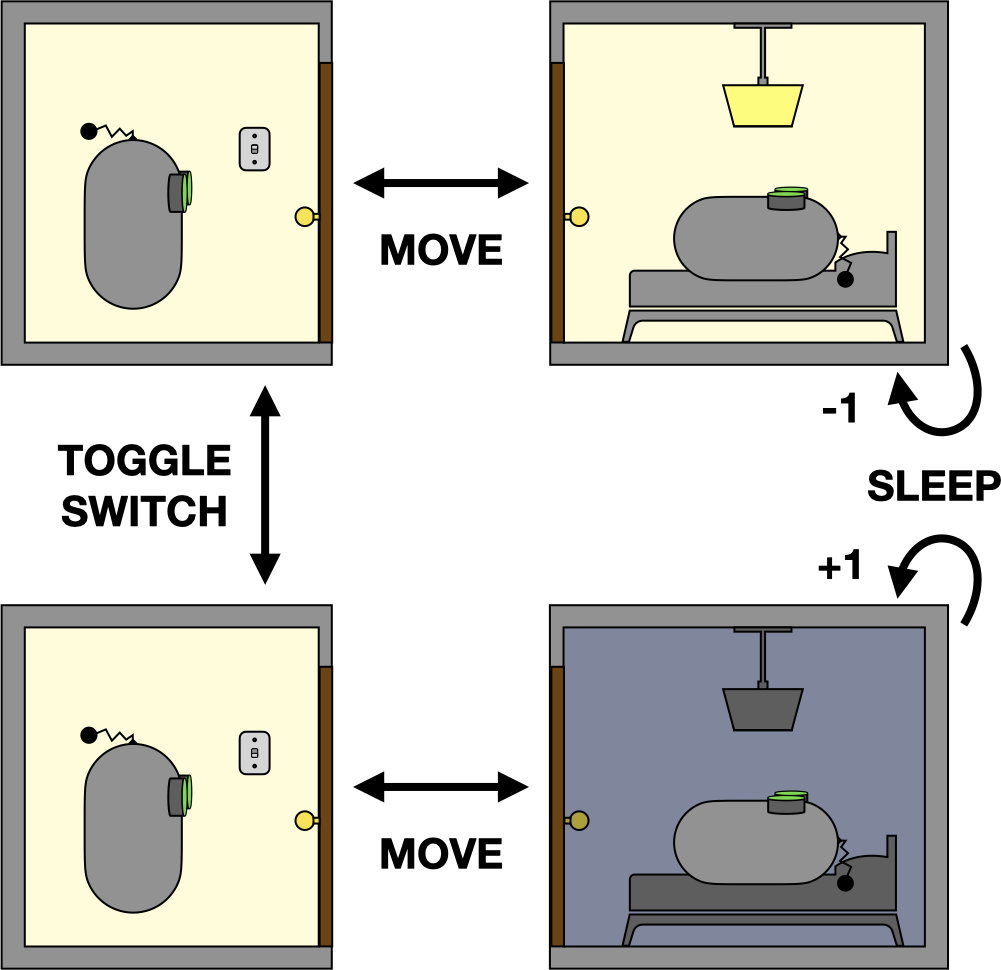Another representation of the bedtime problem.

If the agent’s sensors are precise enough to resolve the position of the lightswitch, then this is a perfectly acceptable alternative (Markov) representation of the problem. However, if the sensors are insufficient to resolve the position of the lightswitch, the decision problem will begin to resemble the following: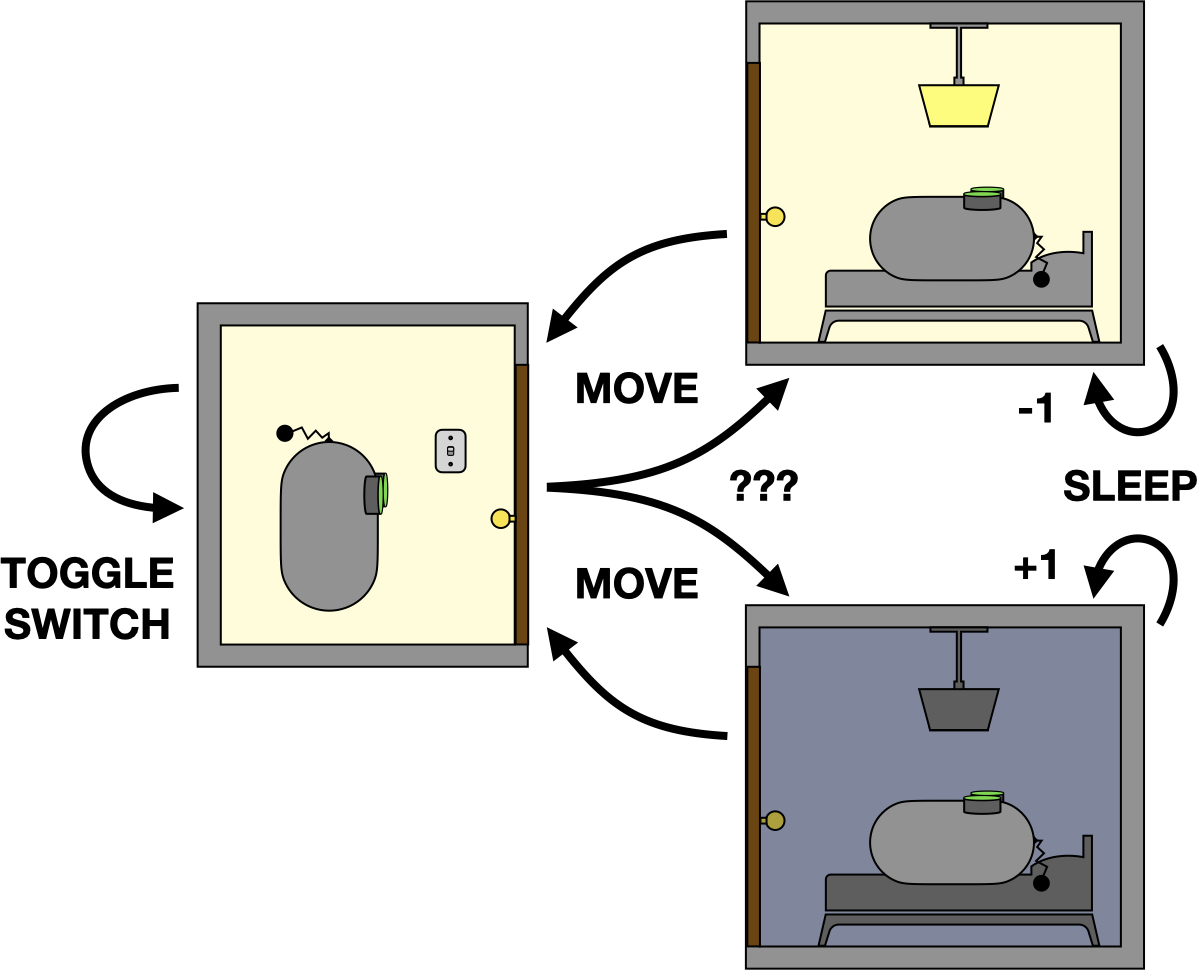A non-Markov representation of the bedtime problem.

Because the agent is no longer tracking the difference between hallway states, it can’t accurately predict the state of the bedroom light. Now, going to bed requires walking into the bedroom to observe the light, potentially returning to the hallway to toggle the switch (while remembering whether the light is on), and finally going to sleep once the light is off.

This notion of remembering things can get quite complicated, since it can involve longer histories than just one step. For instance, if the agent moved from the bedroom to the hallway, then toggled the lightswitch $$N$$ times, subsequently predicting the state of the bedroom light would require maintaining $$N+1$$ steps of history.

When the representation is Markov, an RL agent only needs to consider behaviors that can be expressed as a function of the current state (i.e. Markov policies). But if the representation is non-Markov, the agent may need to condition its behavior on any number of past states and actions, which leads to an exponential increase in the number of possible behaviors it must consider. On the other hand, ignoring history information and simply pretending the representation is Markov (even if it isn’t) can lead to sub-optimal decisions.

## Representation Learning and State Abstraction

One of the central goals of AI is to design general-purpose agents capable of solving a wide range of problems. The “bedtime problem” described above is just one of the many highly important tasks a general-purpose robot may be faced with during its lifetime. A robot may also need to fold laundry, drive a car, or play a game of chess.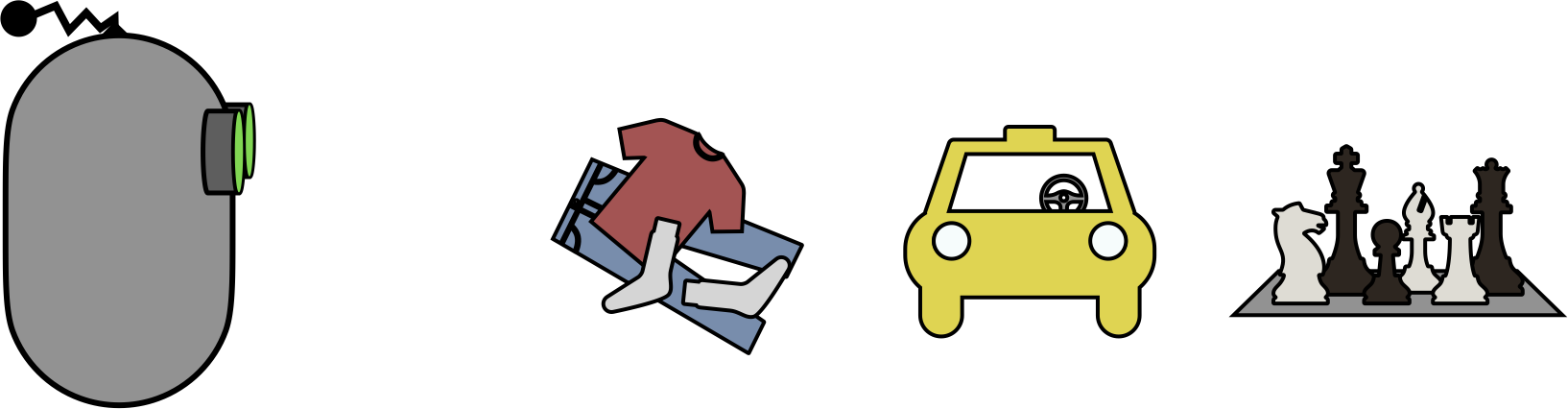A general-purpose robot will face many highly important tasks.

To solve general problems, agents need general-purpose sensors. We’ve already seen how the agent’s sensors affect its representation, and that the representation in turn influences the decision making process. Agents who are likely to encounter lightswitches should be equipped with cameras precise enough to resolve the state of those lightswitches. If those same agents also need to fold laundry and drive cars and play chess, the cameras must support those behaviors as well.

Unforunately, general-purpose sensors introduce new challenges for effective decision making. Rather than making decisions in the appropriate problem-specific representation, the agent must make decisions using its problem-agnostic sensors. For example, rather than learning the optimal policy for a bedroom MDP conditioned on one of just four possible world-states, a general-purpose agent must instead condition its behavior on the limitless number of possible images produced by its high-resolution camera.

To enable efficient decision-making in the face of rich observations from general-purpose sensors, we will turn to state abstraction. In general, abstraction is the process of summarizing information or computation to reveal its essential structure, typically for the purpose of generalization. In the context of decision-making, a state abstraction is a function $$\phi$$ that maps from a rich sensor observation $$s$$ to a simpler, problem-specific abstract representation $$z = \phi(s)$$, with the hope that decision-making is easier when conditioned on the abstract representation $$z$$. By replacing $$s$$ with $$z$$, we can generalize over a potentially infinite number of rich observations using a single abstract state.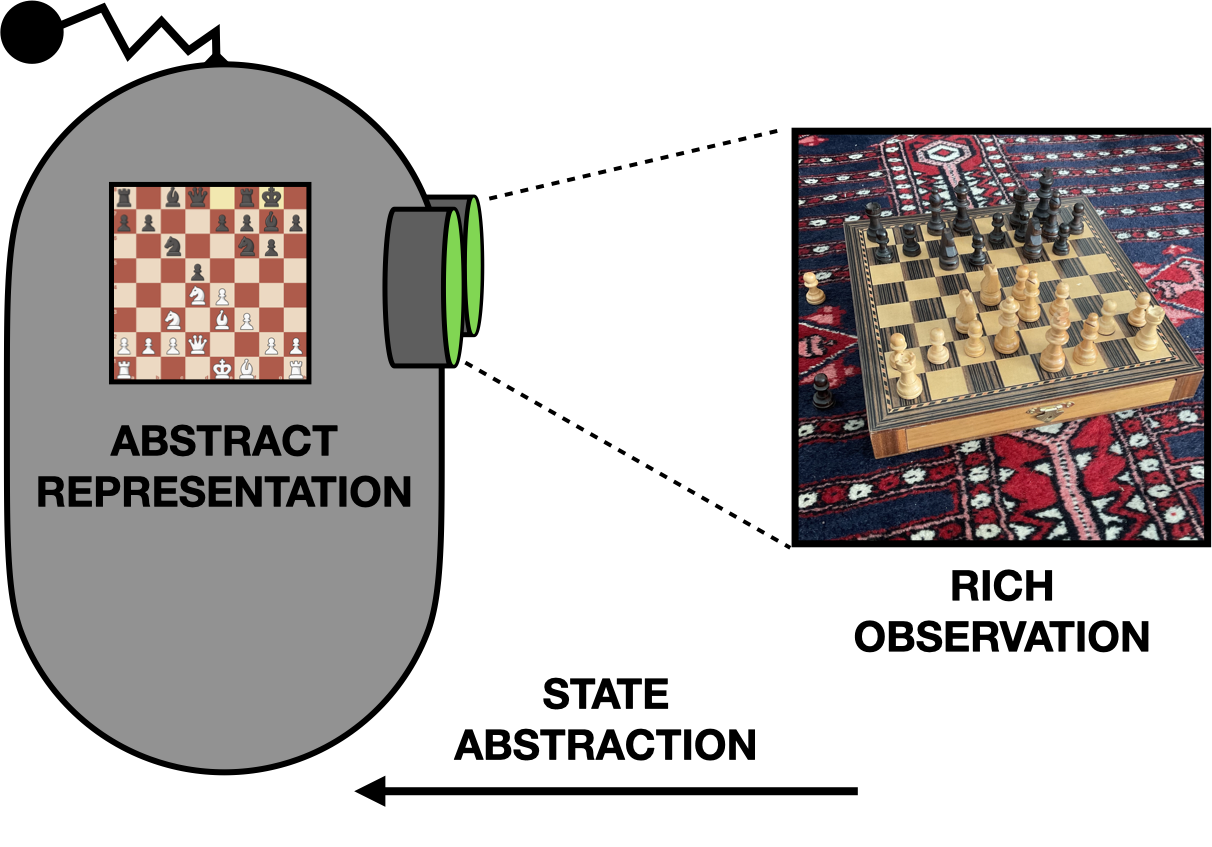A state abstraction for playing chess from image observations.

By definition, general-purpose sensor observations contain too much information for any specific task. When learning a problem-specific abstraction, the goal is to determine which information is essential for solving the problem and which can be safely thrown away. For example, can the chess abstraction ignore the pattern on the carpet? Can the bedtime abstraction throw away information about the lightswitch, or will that cause the representation to lose the Markov property?

In this work, we introduce novel theoretical conditions that guarantee a state abstraction preserves the Markov property, provided that the rich observations themselves were a Markov representation to begin with. We then adapt those conditions into a practical training objective for learning such an abstraction directly from the agent’s experiences.

## Markov State Abstractions

Our main result is that a state abstraction $$\phi$$ is Markov if both of the following conditions hold:

1. We can explain what action (or distribution over actions) caused every state transition $$s \rightarrow s'$$, and the explanation doesn’t change if we instead consider abstract state transitions $$\phi(s) \rightarrow \phi(s')$$.

2. We can distinguish real state transitions $$s \rightarrow s'$$ from made-up state transitions $$s \rightarrow \tilde s'$$ (which may be either valid or impossible), equally well regardless of whether we look at the original states $$s$$, or the abstract states $$\phi(s)$$.

In other words, if the abstraction preserves enough information that we can do both of these things in the abstract decision process, then the abstraction also preserves the Markov property, and we can use it for abstract decision making. In the full paper, we formally prove that these two conditions are sufficient for an abstraction to preserve the Markov property.

To illustrate this point, we can return to the non-Markov representation of the bedtime problem from earlier. One way to look at the three-state representation is that it’s an abstraction of the four-state version. The abstraction ignores the lightswitch and thereby groups the hallway observations into a single abstract state: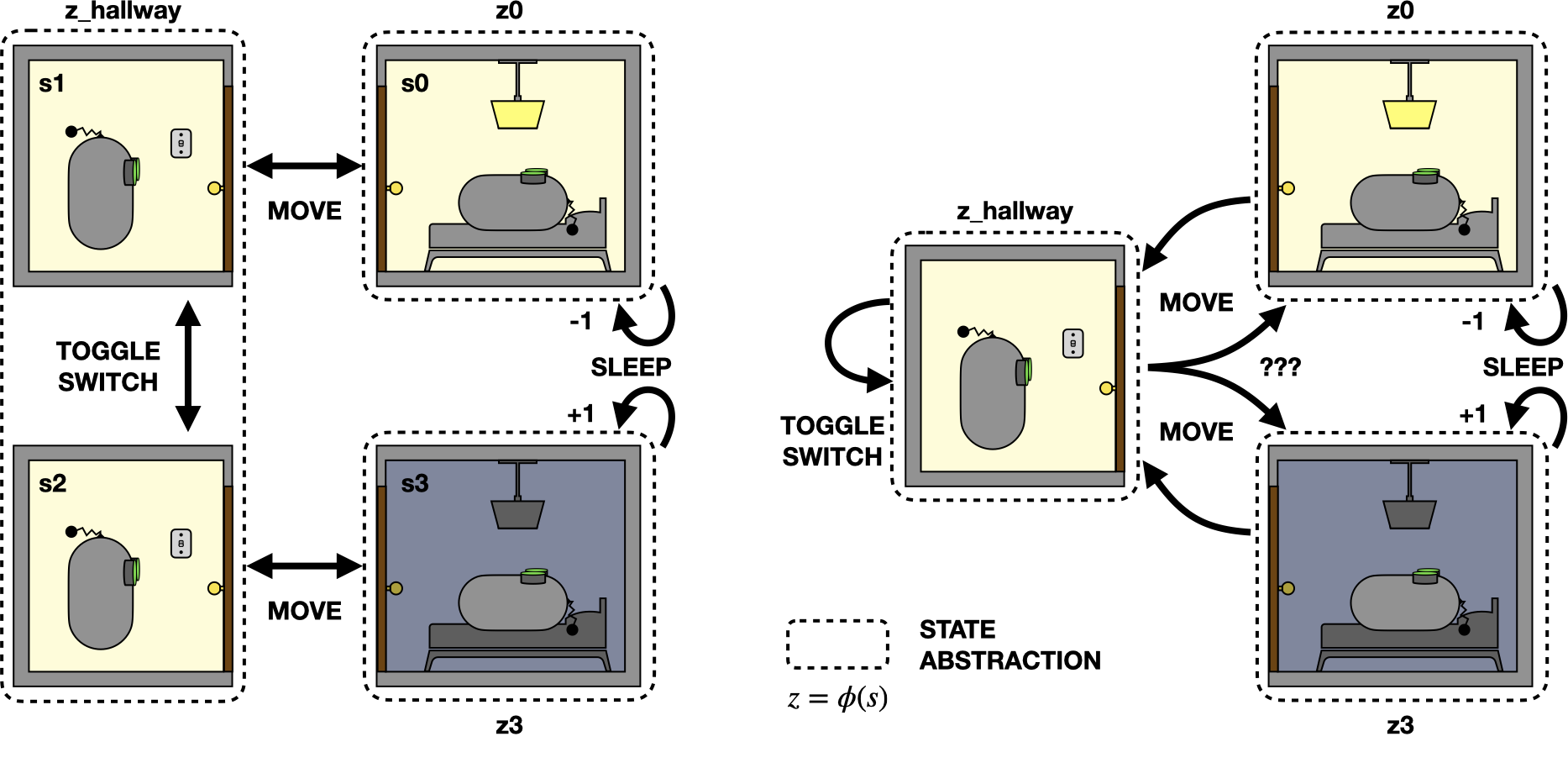A non-Markov abstraction of the bedtime problem that combines hallway states.

We can check whether the two conditions outlined above hold for this abstraction. The first condition involves explaining every state transition. All of the actions can be uniquely identified by the state transition they produce, regardless of which representation we use. For example, the only way to observe a state transition from the hallway to the bedroom is with the MOVE action, and the only way to observe a transition that both starts and ends in the hallway is with the TOGGLE_SWITCH action. Thus, we can explain every state transition equally well using either representation, and the first condition is satisfied.

However, this abstraction violates the second condition about distinguishing real and fake state transitions. Notice that the MOVE action is well defined for the original four-state MDP but that it has ambiguous results under the abstraction. So with this abstraction, we cannot distinguish real state transitions from fakes when they go from the hallway to the bedroom.

## Training Architecture

The two conditions from the previous section are useful for checking whether a given abstraction is Markov, but they are not expressed in a useful format for learning such an abstraction. We therefore derive optimization objectives suitable for training a Markov abstraction with neural networks. After converting each of the theoretical conditions into an optimization objective, we can then train an abstraction in conjunction with solving the resulting optimization problem, and thereby encourage the learned abstraction function to be Markov.

Condition 1 involves a form of “inverse dynamics model”. We typically think of an MDP as having forward dynamics that we can express as a distribution $$\Pr(s'\vert s, a)$$ over possible next states $$s'$$ given a particular state-action pair $$(s, a)$$. By contrast, an inverse model predicts the action distribution for a given state-and-next-state pair: $$\Pr (a\vert s', s)$$.

Condition 2 is a type of “contrastive” or “discriminative” model, since it is deciding between two different types of inputs: real state transitions and fake ones. In other words, it can be implemented as a simple binary classifier.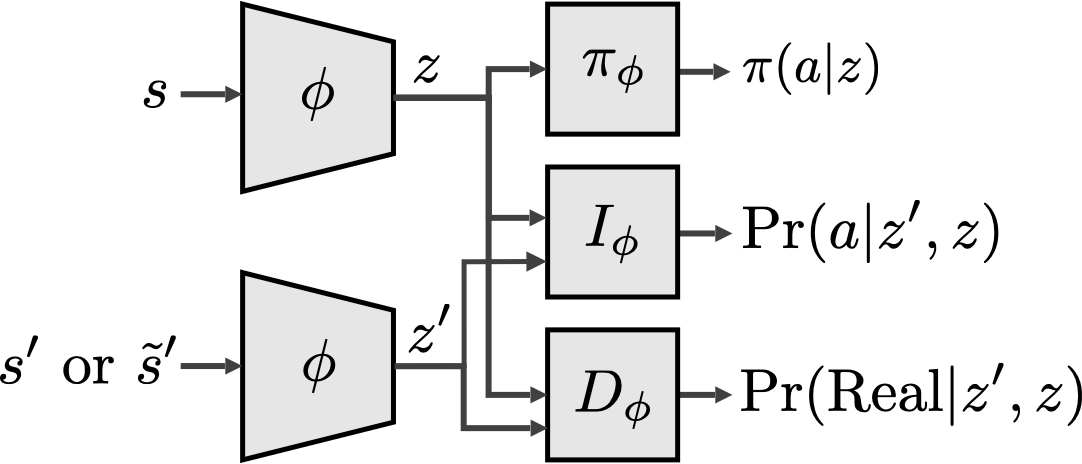Architecture for training a Markov state abstraction.

To learn a Markov state abstraction we train a neural network to do both jobs at once. The original MDP states $$s$$ are converted using the abstraction $$\phi$$ into abstract states $$z$$, where $$z=\phi(s)$$. The resulting abstract states are then used to train an inverse model $$I_\phi$$ and a discriminator $$D_\phi$$. The inverse model learns explanations for abstract state transitions, while the discriminator learns to predict which state transitions are real and which ones are fake.

Since the inputs use the original MDP state representation $$s$$, but the predictions depend only on the abstract representation $$z$$, we are able to train the abstraction such that we can explain and distinguish state transitions equally well using the abstract representation. This allows decision making to take place at the abstract level: the policy $$\pi$$ depends only on the abstract state $$z$$.

The training architecture allows us to encode the Markov conditions as a practical neural network training objective, thereby expressing our theoretical constraints as an optimization problem for directly incentivizing learning a Markov abstraction.

## Gridworld Experiment

Earlier, we talked about how general-purpose agents require general-purpose sensors, and how those sensors contain more information than is sometimes useful for decision making. To test our training architecture on the type of general-purpose sensors that we care about, we will use a visual gridworld navigation problem. The agent can move horizontally or vertically to any of the discrete grid positions, but its observations come from a noisy camera positioned above the grid.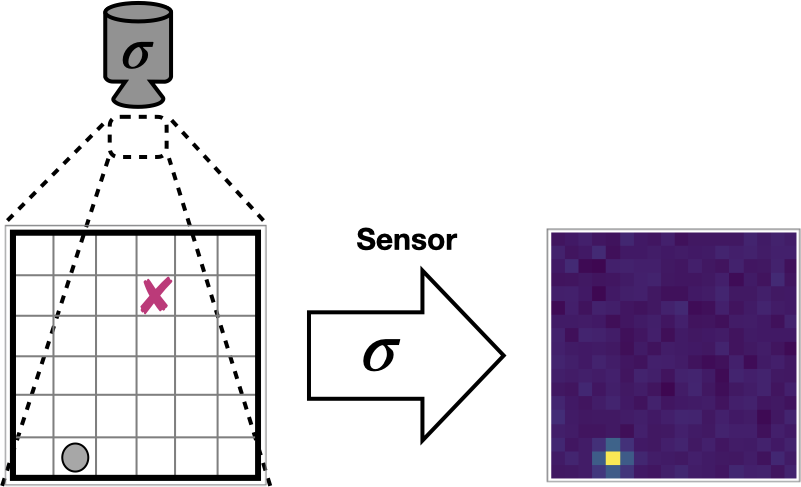A gridworld navigation task with visual observations.

The objective is to navigate to a particular location (marked here with an X). The agent receives -1 reward for every timestep until it reaches the goal, at which point the episode ends, then the agent respawns in a randomly chosen location and the process repeats.

We can see exactly how important it is to choose the right representation for the gridworld problem if we compare decision making with visual features vs. with “expert” features describing the precise x-y grid position (which normally would be hidden from the agent).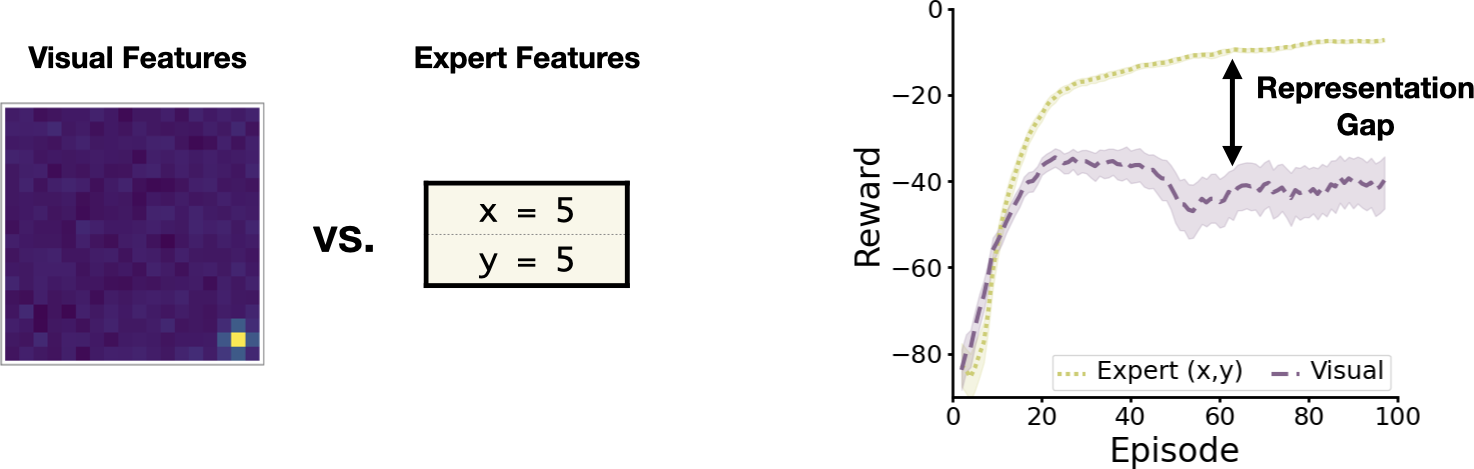The gap in learning performance for two different gridworld representations.

Clearly there’s a significant gap in learning performance between the two representations. The hope is that state abstraction can help us close the gap. If we can learn an abstract representation that recovers the underlying structure of the gridworld, maybe the agent will be able to learn more efficiently.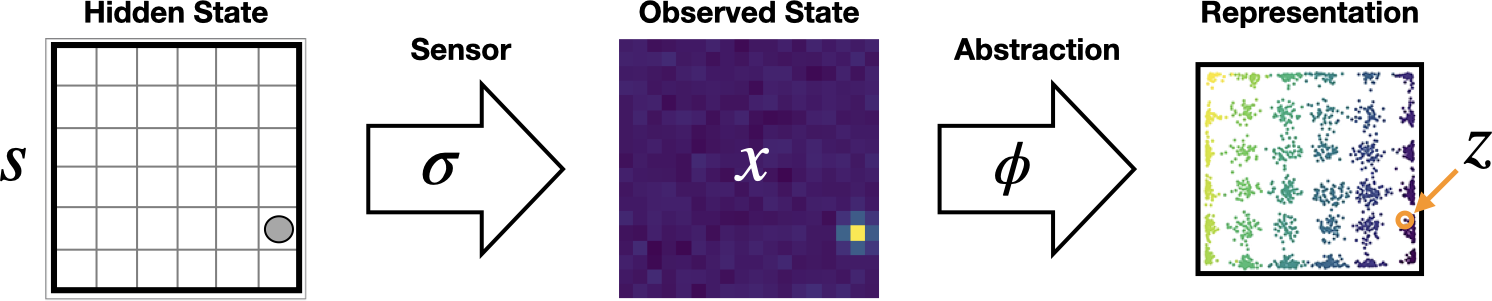The state abstraction pipeline.

We will test our approach as follows. We allow the agent to randomly explore the gridworld and generate experience data (ignoring goal/reward information for now), then we use those experiences to train a Markov state abstraction using the architecture from the previous section.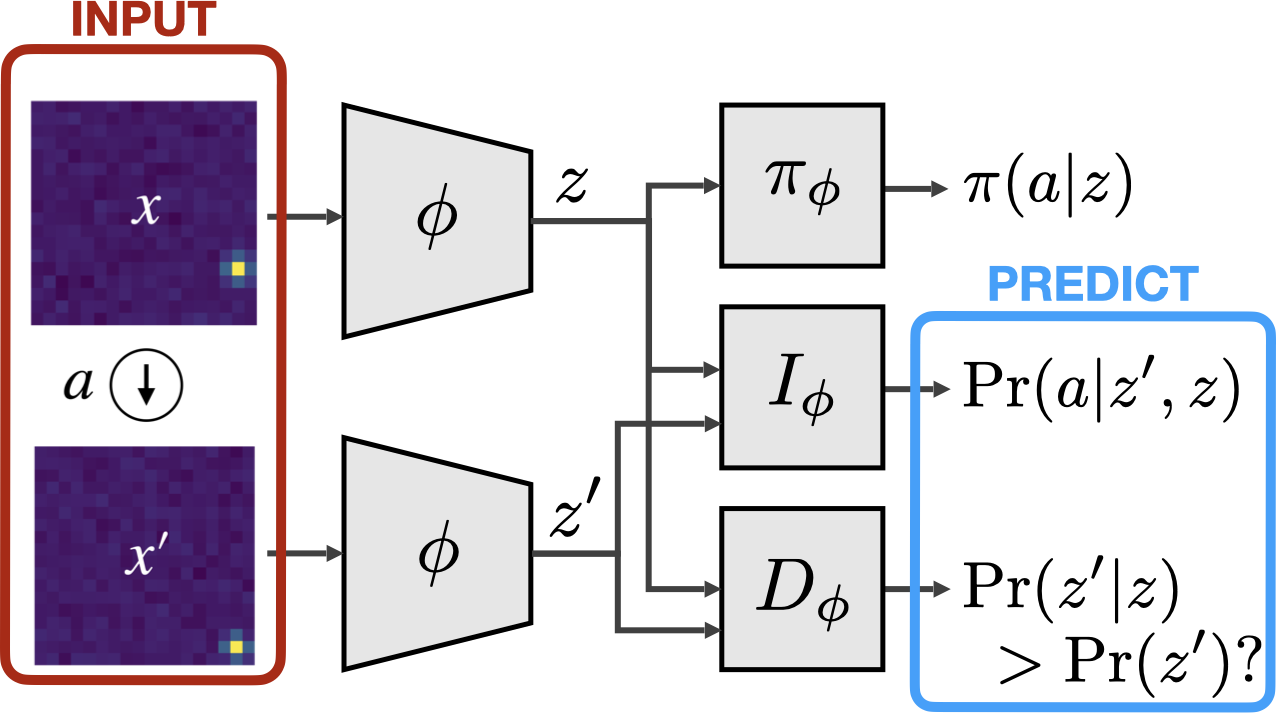Applying the Markov state abstraction architecture to the gridworld problem.

We constrain the learned representation $$z$$ to be two-dimensional, so it’s easier to visualize.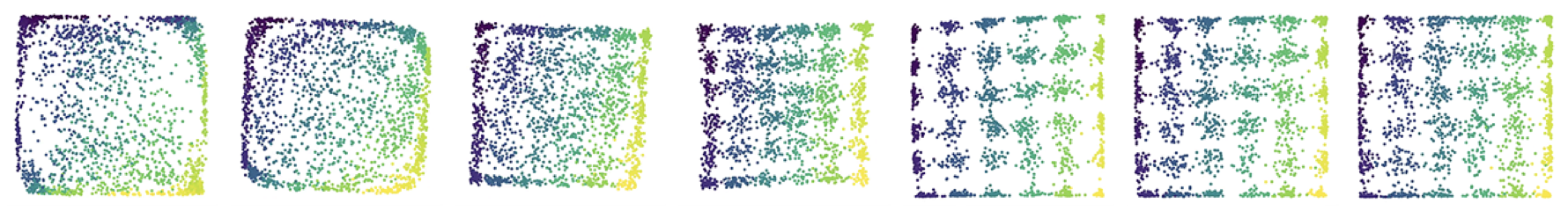Training progress (left to right) of a 2-D Markov state abstraction.

As training progresses, the representation quickly converges to one that reflects the underlying grid structure. The color of the points here denotes the hidden “expert” x-y position and is not shown to the agent. This demonstrates that the agent is able to discover the problem-specific structure.

Better still, if we use the resulting representation for decision making, we’re able to fully close the representation gap!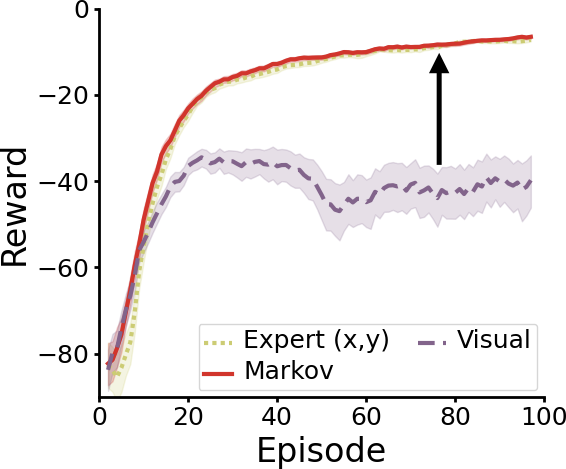Learning performance with a Markov state abstraction.

The red line shows reinforcement learning performance when using the learned Markov representation, which turns out to be just as good as with the expert features. Essentially, our method allows the agent to autonomously construct its own problem-specific abstract representation, then use that representation for decision making instead of its built-in sensor observations.

## Simulated Robotic Control

To test the generality of our approach, we next looked at a set of simulated robotic control tasks from the DeepMind Control Suite. These tasks involve controlling a simulated robot in order to perform various activities from rich, visual observations.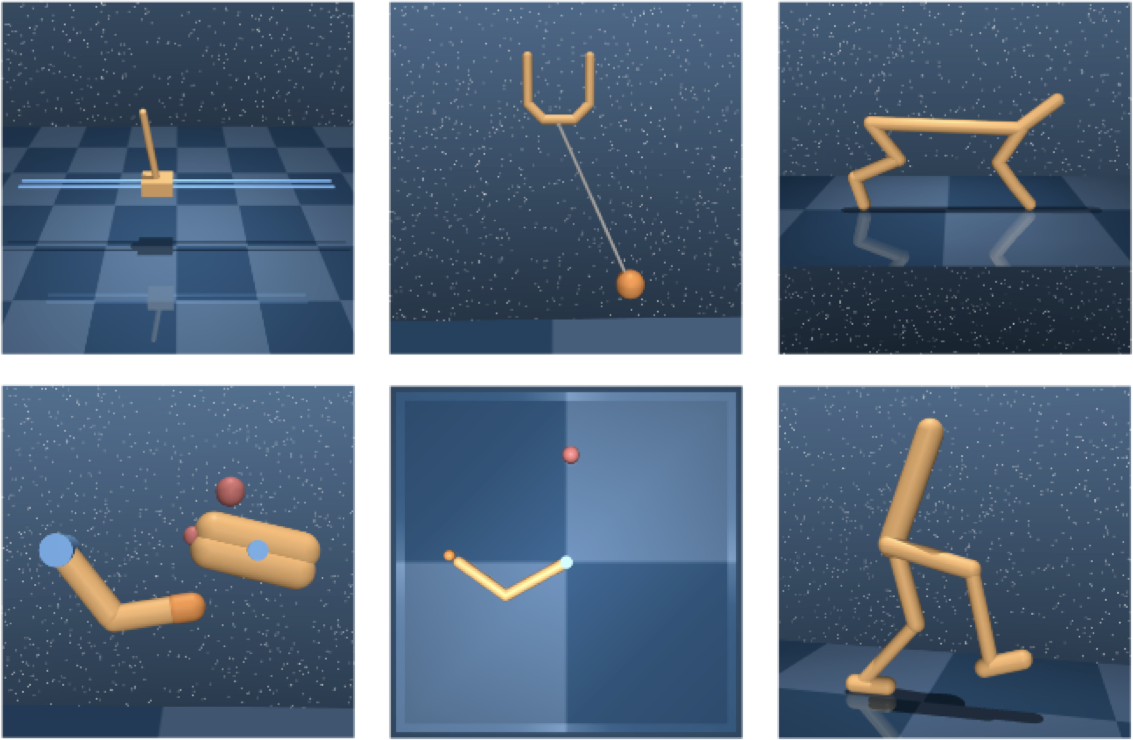Example observations from six tasks in the DeepMind Control Suite.

We again train a Markov state abstraction, and compare our method against several other representation-learning approaches.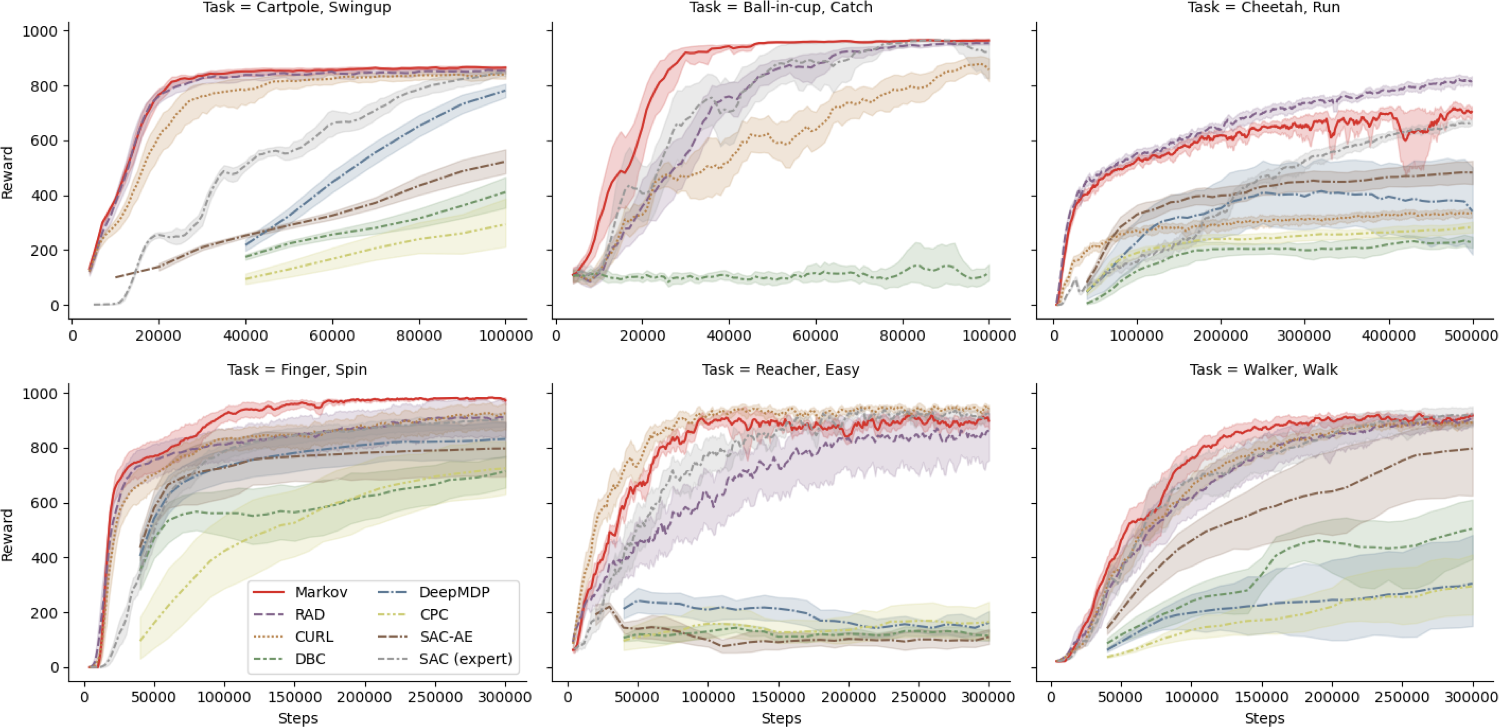Learning performance on the DeepMind Control Suite.

We find that Markov state abstractions (in red) achieve substantially improved learning performance over a baseline algorithm (the “RAD” agent, in purple) that does not attempt to learn such an abstraction. We also find that the resulting agent beats every other baseline in a head-to-head comparison on the majority of domains.

## Wrapping Up

We started by introducing Markov decision processes (MDPs) as a useful way to formalize the reinforcement learning problem. We talked about how choosing the right problem-specific representation is important for efficient decision-making, and saw that modeling a problem as an MDP requires a Markov state representation. We also saw that while state abstraction can be helpful for making decisions when faced with general-purpose sensors, it unfortunately isn’t guaranteed to produce a Markov representation.

To overcome this issue, we outlined a set of theoretical conditions that are sufficient for an abstraction to preserve the Markov property, and introduced a practical training objective for learning such an abstraction from data. We demonstrated that the resulting approach is able to learn a representation of a visual gridworld problem that recovers the underlying grid structure and that fully closes the representation gap, matching the learning performance of using expert features. Finally, we showed that our approach leads to state-of-the-art representation learning performance on a variety of simulated robotic control tasks.

For a more detailed discussion, including a formal proof that the two conditions we introduce are sufficient for a Markov abstraction, plus an in-depth derivation of the training objectives, check out the full paper. We also discuss a number of alternative methods for learning Markov state abstractions, and include a few more experiments in the appendix.